# MCAT Physical : Acid-Base Reactions

## Example Questions

### Example Question #1 : Acid Base Reactions

HCN dissociates based on the following reaction.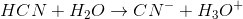The Ka for hydrogen cyanide is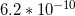.

Suppose that a solution with a pH of 4.5 has 2M HCN added. Which of the following values will change?

Kw

Ka

pOH

Kb

pOH

Explanation:

Remember that equilibrium constants are not affected by the concentrations of the reactants and products. Since an acid is being added to the solution, the pH of the solution will be affected. This means that the pOH will be affected as well.

### Example Question #1 : Acid Base Chemistry

100mL of an unknown solution of NaOH is titrated with 3M HCl until neutralized. The resulting solution is evaporated, and 3.0g of white crystal are recovered. What was the concentration of the NaOH solution?

0.5M

0.3M

0.7M

1.25M

1.0M

0.5M

Explanation:

In the neutralization reaction between NaOH and HCl, NaCl salt is formed. When the solution is evaporated, this salt is left behind.

3.0g of NaCl is equivalent to 0.05mol NaCl. Since the titration is between a strong acid and a strong base, all of the NaOH in the original solution is converted to NaCl in a one-to-one ratio, meaning that mol NaCl = mol NaOH.

We now know that there was 0.05mol NaOH in the 100mL solution, so the concentration must have been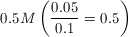.

### Example Question #25 : Acid Base Chemistry

How many milliliters of 0.05M HCl are required to neutralize 200mL of 0.025M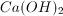?

400mL

200mL

500mL

100mL

250mL

200mL

Explanation:

First note that there are two moles of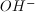for each mole of.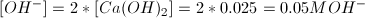Then calculate the number of moles ofin the given volume of solution.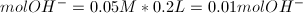To neutralize, we need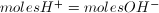.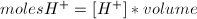We can plug in our value of 0.01mol and the given concrentration of 0.05M, and solve for the required volume.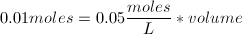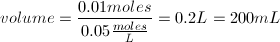### Example Question #6 : Acid Base Chemistry

What volume of 0.375M H2SO4 is needed to fully neutralize 0.5L of 0.125M NaOH?

0.5L

41.7mL

1.5L

83.3mL

167mL

83.3mL

Explanation:

This question requires use of the simple titration equation M1V1 = M2V2. The key is to identify that sulfuric acid has two equivalents of acidic hydrogens while NaOH has only one hydroxide equivalent. All wrong answer choices result from making this mistake or other calculation errors.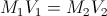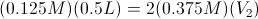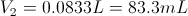### Example Question #26 : Acid Base Chemistry

Which of the following is true regarding an acid and its pKa?

I. One can increase the strength of an acid by decreasing its pKa value

II. pKa increases as the acid dissociation constant decreases

III. pKa of an acid cannot be changed by altering the concentration of the acid

I, II, and III

I and III

II and III

I and II

II and III

Explanation:

Recall that pKa is defined as follows: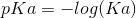Here,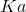is the acid dissociation constant.is a measure of the equilibrium strength of an acid and is unique for each acid. The higher the value of, the stronger the acid; however, a particular acid’svalue, and subsequently its strength, can never be changed. The only way you can change theof an acid is by changing the identity of the acid itself. This means that the pKa value of an acid is also always constant; therefore, you cannot decrease an acid’s pKa.

Using the definition of pKa, we can see that the pKa of an acid increases as you decrease the acid dissociation constant (). A strong acid will have a highand a low pKa.

The pKa of an acid can never be altered; therefore, changing the concentration of the acid will not alter the pKa of the acid. It might change the amount of hydrogen ions produced and alter the pH; however, the pKa of the acid will stay constant.

### Example Question #27 : Acid Base Chemistry

Consider two solutions: solution A and solution B. Solution A is a 0.1M hydrogen iodide solution and solution B is a 0.1M hydrochloric acid solution. What can you conclude about these two solutions?

Solution B has twice as many hydrogen ions as solution A

Both solutions have high pKa values

Both solutions have approximately equal amounts of hydrogen ions

Solution A has twice as many hydrogen ions as solution B

Both solutions have approximately equal amounts of hydrogen ions

Explanation:

To answer this question you need to write out the acid dissociation reaction for hydrogen iodide and hydrochloric acid.

The acid dissociation reaction for hydrogen iodide is: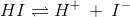The acid dissociation reaction for hydrochloric acid is: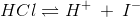Recall that both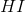and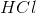are very strong acids; therefore, they will dissociate completely in solution and produce their respective products. Since the ratio of acid to hydrogen ions is 1:1 for both acids AND the concentration of both acids is the same (0.1M), the amount of hydrogen ions produced will be the same for both solutions.

Recall that pKa decreases as the Ka (acid dissociation) increases. As mentioned, both acids are very strong; therefore, they will have very high Ka values and, subsequently, very low pKa values.

### Example Question #2 : Acid Base Reactions

__________ the pH of an acidic solution will __________ the pKa of the acid.

Decreasing . . . not change

Decreasing . . . increase

Increasing . . . increase

Increasing . . . decrease

Decreasing . . . not change

Explanation:

The acidity of the solution results from the amount of hydrogen ions present in the solution. We can increase or decrease the pH of the solution by decreasing or increasing the amount of hydrogen ions present, respectively. pKa is a measure of the strength of an acid (meaning how easily it can dissociate into hydrogen ions and its conjugate base). Altering the pH of the solution will have no effect on the strength, and subsequently pKa, of the acid.

### Example Question #91 : Gre Subject Test: Chemistry

Consider the following reaction: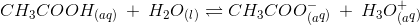Which of the following changes will increase the pH of this solution?

Increasing the pKa of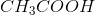Decreasing the volume of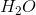Increasing the acetic acid concentration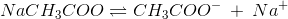The dissociation reaction will produce more acetate ions. According to Le Chatelier’s principle, the increase in acetate ions will shift the equilibrium of the reaction (given in the question) to the left. This means that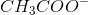and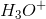will be utilized to form. This will cause a decrease in the amount of hydronium ions in solution. Recall that pH is increased when the concentration of hydrogen ions (or hydronium ions,) is decreased; therefore, adding sodium acetate will increase the pH of the solution.